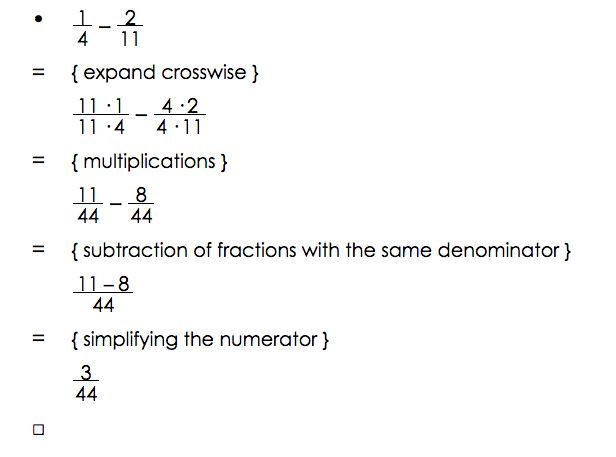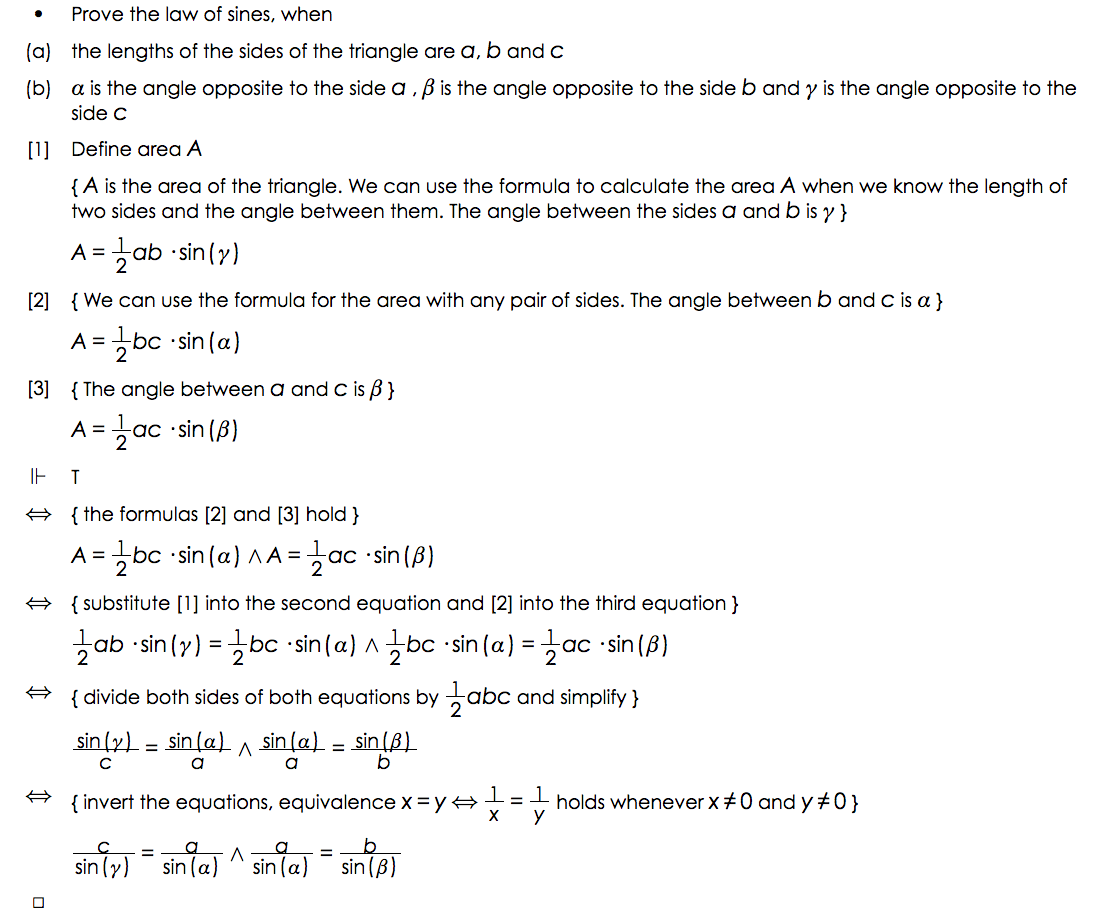# Pedagogy

## Structured Derivations

Structured derivations is a method for presenting mathematical arguments in a precise and easily understandable form. Structured derivations make it easier for students to follow and understand when the teacher presents a mathematical argument. It gives the students a template for how to construct their own solutions to mathematical problems. The uniform presentation format makes it easy to check and find errors in students' solutions. A structured derivation can also be analyzed by a computer,  to check that the derivation is meaningful and that each step in the derivation is mathematically correct.

The format can be used for all kinds of mathematical arguments: calculations, solving equations, simplifying expression, proving theorems, geometrical constructions, and so on. Structured derivations can be used at any level of mathematics, from pre-algebra to university level and research, and can be used in any area of mathematics.

A simple calculation